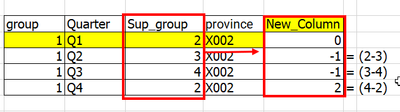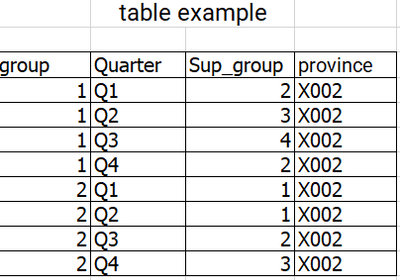cancel
Showing results for
Did you mean:Frequent Visitor

## create a column that is the difference of adjacent variables.

I want to create a column that is the difference of adjacent variables.

Ex. Q1 = Sup_group 2-2 = 0  (Sup_group of Q1 - Sup_group of Q1)

Q2 = Sup_group 2-3 = -1 (Sup_group of Q1 - Sup_group of Q2)

Q3 = Sup_group 3-4 = -1 (Sup_group of Q2 - Sup_group of Q3)

Q4 = Sup_group 4-2 = -1 (Sup_group of Q3 - Sup_group of Q4)

result example.....sample data....thank you,

1 ACCEPTED SOLUTIONSuper User

Hi @only_me321
Please refer to attached sample file with the solution``````Result =
VAR CurrentQuarter = 'Table'[Quarter]
VAR CurrentSupGroup = 'Table'[Sup_group]
VAR GroupProvinceTable = CALCULATETABLE ( 'Table', ALLEXCEPT ( 'Table', 'Table'[group], 'Table'[province] ) )
VAR TableBefore = FILTER ( GroupProvinceTable, 'Table'[Quarter] < CurrentQuarter )
VAR PreviousRecord = TOPN ( 1, TableBefore, 'Table'[Quarter] )
VAR PreviousSupGroup = COALESCE ( MAXX ( PreviousRecord, 'Table'[Sup_group] ), CurrentSupGroup )
RETURN
PreviousSupGroup - CurrentSupGroup``````
2 REPLIES 2Super User

Hi @only_me321
Please refer to attached sample file with the solution``````Result =
VAR CurrentQuarter = 'Table'[Quarter]
VAR CurrentSupGroup = 'Table'[Sup_group]
VAR GroupProvinceTable = CALCULATETABLE ( 'Table', ALLEXCEPT ( 'Table', 'Table'[group], 'Table'[province] ) )
VAR TableBefore = FILTER ( GroupProvinceTable, 'Table'[Quarter] < CurrentQuarter )
VAR PreviousRecord = TOPN ( 1, TableBefore, 'Table'[Quarter] )
VAR PreviousSupGroup = COALESCE ( MAXX ( PreviousRecord, 'Table'[Sup_group] ), CurrentSupGroup )
RETURN
PreviousSupGroup - CurrentSupGroup``````Frequent Visitor

Hi @tamerj1 ,

Thank you for the DAX. It helped me to pass well. 🙏🙏🙏